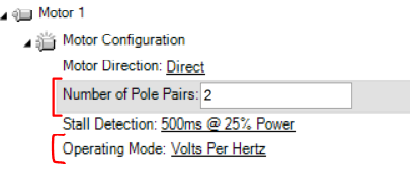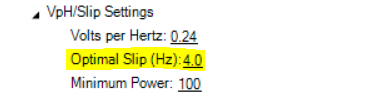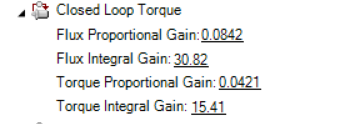AC induction motors are reliable and cost-effective, factors that are very important when picking a fitting motor for an application. In the present guide we are going to set up an AC induction motor on a GIM2660T controller. This guide will serve as an additional help to customers aiming to create an effective PID tuning of their AC induction motor.

An extremely important first step while trying to set up an AC induction motor or any other motor is to have as much information as possible regarding the motor we want to work with. The information could come either from the datasheet of the motor or the label of the motor itself.

In our case our motor has the following characteristics:

• P      = 400 W
• Vbat = 24 V
• I        = 26 IF A
• n       = 2800 RPM
• f        = 100 Hz
• V fase= 3*16
• Enc    = 32 pulses
• Poles = 4

Volts Per Hertz Mode

We first start by working at VPH(volts per hertz) mode, the open loop mode in our Induction Motor firmware. We have to set the pole pairs and the Volts per Hertz. Do not use the encoder as a feedback on this step, it can be configured though.

Volts per Hertz can be calculated by dividing the Phase to Phase voltage Amplitude to the nominal frequency of the motor.

On our case:  24 Volts/ 100 Hz = 0.24 VpHThe motor should be able to run without issues.Optimal Slip Mode

The first closed loop mode of our controllers. It is not used as much as the next two as it is not as efficient.

warning! This is closed loop mode is designed for very simple - low demanding applications. It is not the best approach, as only the slip of the motor is being controller and the motor current can reach undesired values.

In order to continue working from this step onward we need to have additional information about the motor. We need to know the following values:

1. Rs = 24,6 mΩ
2. Rr = 24,5 mΩ
3. Lls = 67 μH
4. Llr = 67 μH
5. Lm= 961μH

For this mode to work we have to first calculate the Optimal Slip. In order to calculate the optimal slip we have to use the formula below.According to the values from our motor, we can calculate the slip. On the particular case the slip will be

S = 24,6 mΩ/(2*3.14*961μH) = 4.0762Additionally, before running the motor we should check, in open loop, that the encoder/feedback has the same polarity as the direction that the motor is spinning.

Finally, we have to tune the motor by setting the closed loop speed PID parameters. As stated in the manual starting from values of P=0, I=1, D=0 is a good starting point.

Below we can see a successful tuning of the motor for closed loop constant slip with P=0, I=0.5, D=0. Of course this case is for a motor spinning free without load. Additional tuning will be needed for cases with load and more complex applications.FOC Torque Mode

Next mode we need to configure in order to reach the FOC speed mode is the FOC torque mode. It is quite important that we set up this mode since this is where we will introduce the FOC parameters to the controller.

First step in this mode is to set the optimal flux Amps. In order to do that, we will need to change the mode to open loop (Volts per Hz) and run the motor with the motors using the rated Volts per Hertz ratio. In this case it is 12.4 A. This flux amps can be increased (Flux Strength) for low speed and high torque operation requirements and can be decreased (Field Weakening) for high speed and low torque operation requirements.So we set the Rotor Flux Current to :12.4 AFollowing that, we need to calculate the FOC parameters. Bandwidth is in rad/sec and according to Nyquist criteria the current loop bandwidth cannot be more than the half of the current loop sampling time. Most commonly the current loop bandwidth is set to the 1/10-1/20 of the current loop sampling time. The current loop sampling time is at 1kHz.

We have all the data from the motor available so we are able to do that easily by using the appropriate formulas.

Below, finding the gains for 50HZ Bandwidth. Usually even smaller bandwidth can be as effective as the 50Hz. It is better to start with the smaller possible gains and then tune according to the behavior of the motor.

Torque FOC Kp : ( Llr + Lls ) x 2π x BandWidth = 67μH + 67μH * 6.28 * 50 = 0.0421

Torque FOC Ki : ( Rr + Rs ) x 2π x BandWidth = 24.5mΩ + 24.6mΩ * 6.28 * 50 = 15,41

Flux FOC gains should be 2x the values of the Torque FOC gains.

Flux FOC Kp : 2 * 0.0421 = 0.0842

Flux FOC Ki  : 2 * 15.41   = 30,82Warning! Test in FOC torque mode with caution.

FOC Speed Mode

It is vital to have completed all the steps above in FOC torque mode in order to achieve a good result in FOC speed mode.

Additional to the steps of the FOC torque mode, we need to introduce some Closed Loop Speed Gains. These gains should be quite small as a starting point, as by adding the FOC current loop gains, we have a cascaded system.

In my example, I used P 0.02 and I 0.1 which are quite low values.The result is as below, the response while a bit slow and with a slight overshoot, is good and rests on the setpoint. With more tuning this could be improved to a better and more accurate response, according to the requirements of the application.